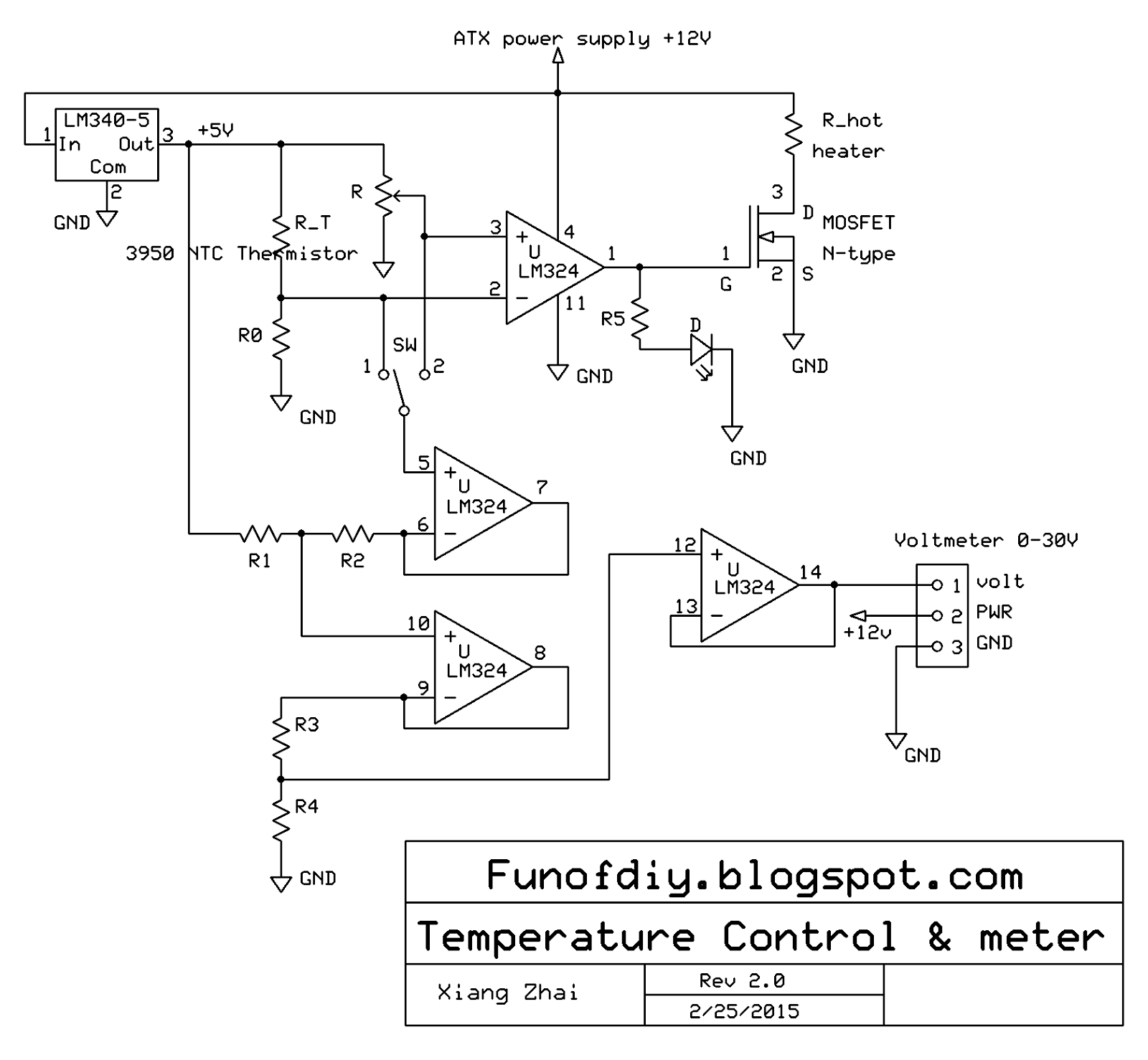## Wednesday, February 25, 2015

### 3D printer hot end temperature control system Version 2 [unfinished]

Almost a years ago, I post the first version of a simple temperature control system ultilizing only a LM324 quard op-amp with some resistors and a MOSFET. The system is for 3D printer's hot end.

The controller has two major functions: (a) turning a power resistor on and off automatically to maintain a constant temperature (adjustable manually); (b) showing the real time temperature in the range of 120-260 C. It is simple, low price, and efficient. The circuit board is shown below.Temperature control system Version 1. See this post for detail. The values of the resistors here are R0=600 ohm, R1=10k ohm, R2=3.9k ohm, R3=6.19k ohm, R4=2.4k ohm, R5=10k ohm.

However, I realized later, also as reader epineh pointed out, the second function, displaying real time temperature, requires a constant +12V power supply. This could be problematic. Because most time people use low price ATX computer powersupply to power their 3D printer. And those powersupply DOES NOT output constant voltage. When there is a large current drain change, say once the power resistor is switched on and off, the power supply's output voltage can jump between 10 and 12V. As a matter of fact, the displayed voltage can be off by 20%!! The control system, on the other hand, works just fine because it relies only on the ratio of the resistance of thermistor and R0.

One solution is to use a logic power supply that output constant 12V for the LM324 and ATX powersupply for the hot resistor R_hot. Or another way is to add a voltage regulator, which turns the unstable 12V to a stable 5V as the voltage reference for LM324. Only one power supply is required. The circuit diagram is shown below.Temperature control system Version 2. The values of the resistors here are R0=600 ohm, R1=10k ohm, R2=3.9k ohm, R3=6.19k ohm, R4=12.4k ohm, R5=10k ohm.

There are two differences between version 1 and version 2.
(a) In version 2, a LM340T5 +5V voltage regulator is used to convert 10~12V to a constant +5V. So the display will not be affected by the status of the hot resistor.
(b) R4 is changed from 2.4k ohm to 12.4k ohm. This is important in order to correctly display the temperature. I will show why later.

[this post is unfinished yet]

1.Thanks for sharing this informative post!
environmental test chamber
Altitude chamber
Salt spray chamber

2.3.4.